New update is available. Click here to update.

# Sum of Digits of a Number in C

## Introduction

Hello Ninja,
Getting started with C Language is the first ladder to becoming a good programmer. This is said because the C language is an elementary language with many theoretical concepts that develop your base for future coding or programming.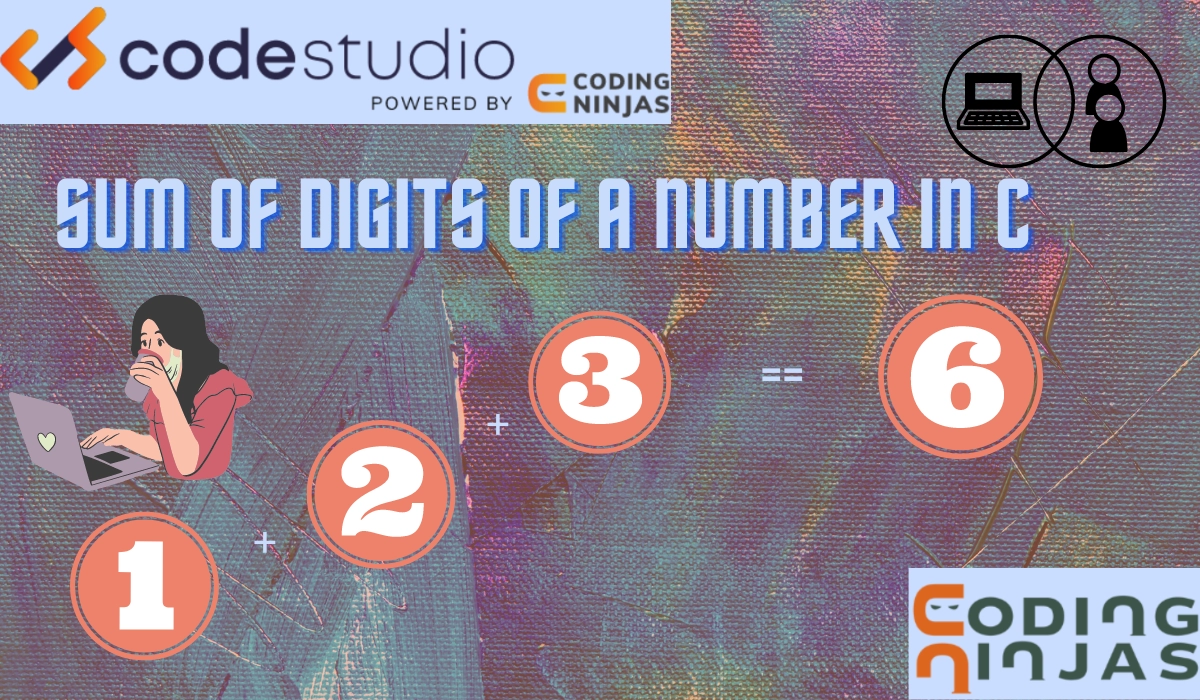Today we will learn the most common type of question in C, the sum of digits in C language. This is the first and the basic program almost everyone has to do to start with the coding and maintain the coding pace in C language.

The C language is one of the most popular programming languages. It is widely used and promoted to learn by various professors as the first programming language. This is because the C language consists of many basic theoretical concepts, which helps create a strong base for future coding or programming.
It is an open-source programming language and is procedural-oriented. It works primarily on functions. In C language, there is full use and reuse of data. The language being a procedural programming language, provides no data hiding.
Dennis Ritchie created the c language in the 1970s. As of today's date, the C language has successors in the technical market.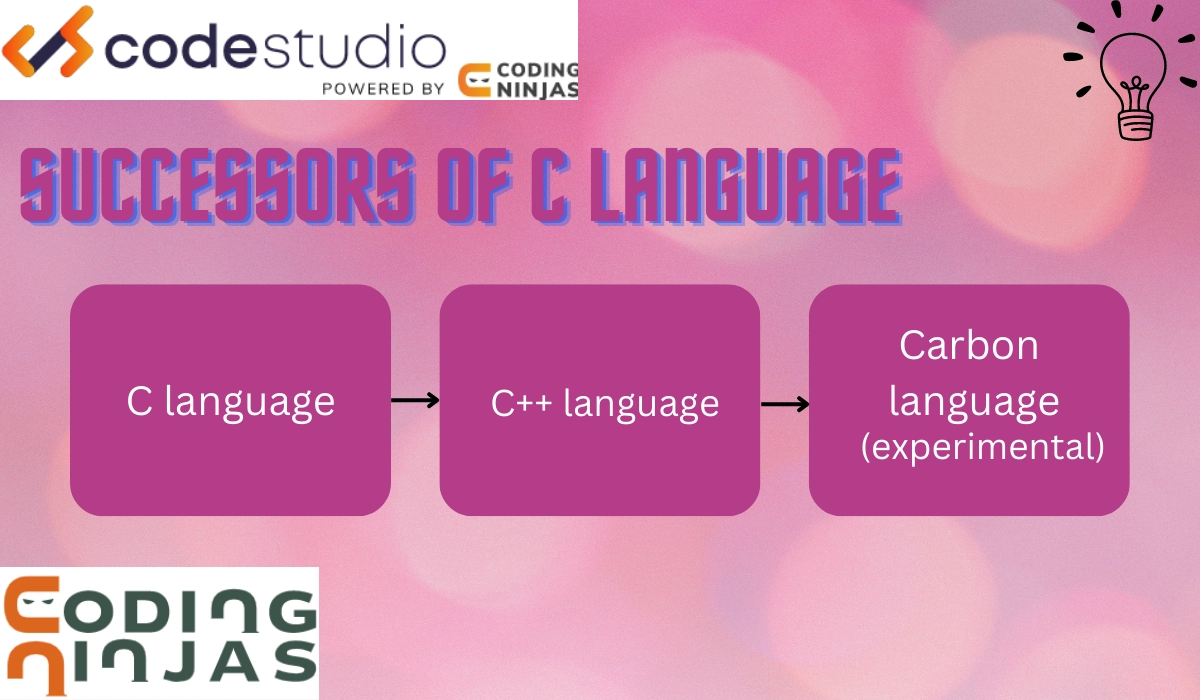We will start you with the C language programming using the example of the sum of digits in C. We will learn different ways to do the sum of digits in C.

## C Language Basics

To write a basic C program such as the one we will discuss in this article. We need some basic knowledge of concepts of the C language.
Whenever we write a program as a beginner or when we are solving big problems, there are a few steps we need to follow.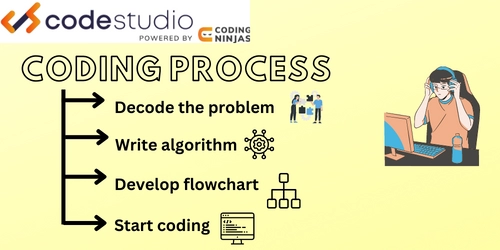• Understand the problem.

• Develop an algorithm - step-by-step instructions to solve a problem.

• Create a flowchart - a pictorial representation of an algorithm.

• Start Coding.

This is how we solve any problem in any programming language. In C language, the basic components of a code are:

• Preprocessors - Add hash(#) included to link the libraries to be used.

• The main function - contains the body of the program.

• Operators - These are symbols that perform special actions in C language.

• Data types - Defines what type of data is given and assigns storage space accordingly. For example, the integer is denoted by int and has a storage space of 2 bytes with integers as its values.

• Expressions - is a statement where operators link operands. For example, x + y, where x and y are operands, and + is an operator.

• Printf(“<statement>”) - prints the statement inside inverted commas on the output screen.

• Scanf() - used to get input from the user.

• Loop - It is used to repeat the action several times. We have a while, do while, for statements for looping.

• Functions are code blocks declared and defined differently(not inside the main function) and are called inside the primary function(main body of the program).

Now that you know the essential components of C and their uses. It will be easier for you to understand the programs we discuss to solve the sum of digits in C.

Also see, Binary to Hex Converter

## Sum of Digits in C

To solve any problem in any programming language, the first step is always to understand the problem thoroughly, as discussed in the previous section. So our problem here is that we have to find the sum of digits in C. Let's suppose a number 257, and we have to perform the sum of digits of a number in C. This means that 2+5+7 is to be done. Keep in mind the difference between sum of numbers and sum of digits in C.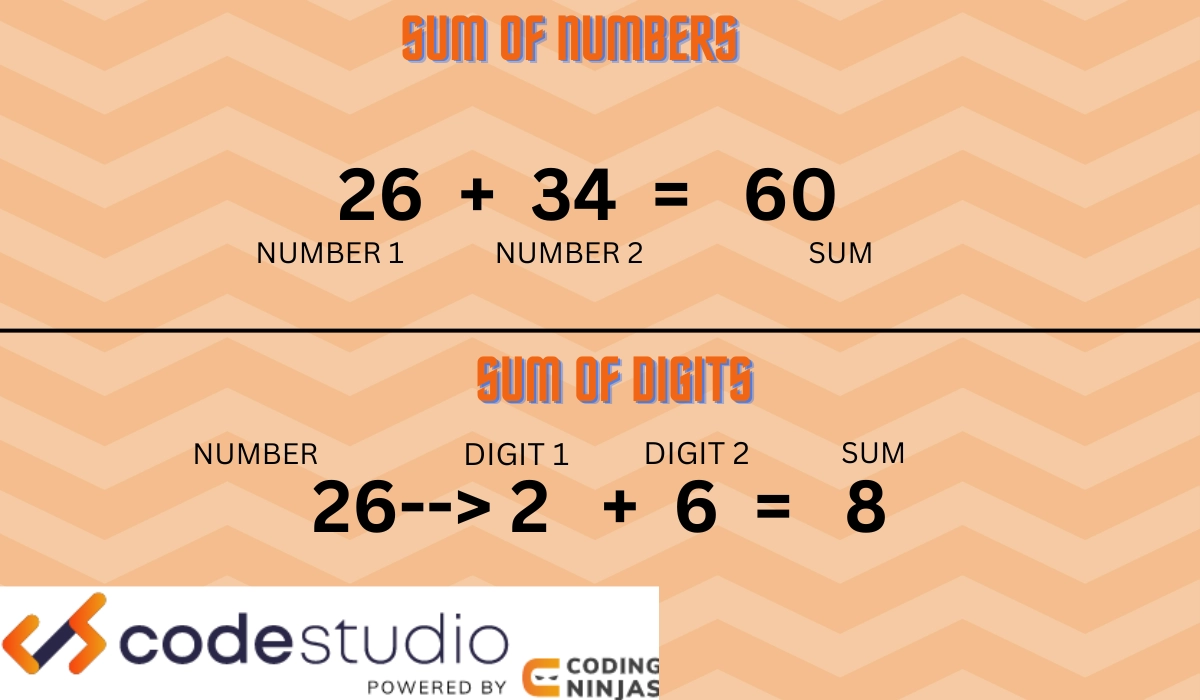We can perform the sum of digits of a number in C in several ways. We will discuss all the programs that can be used to execute the sum of digits of a number in C.

### Program Using While Statement

We can use looping to find the sum of digits of a number in C. For this example, we will use while statements. While the statement has a condition that determines when the loop is to be terminated. It contains a body that runs till the condition is satisfied. We use the modulus operator (%), which returns the remainder in this program.

The picture below shows a flowchart of how the program is executed.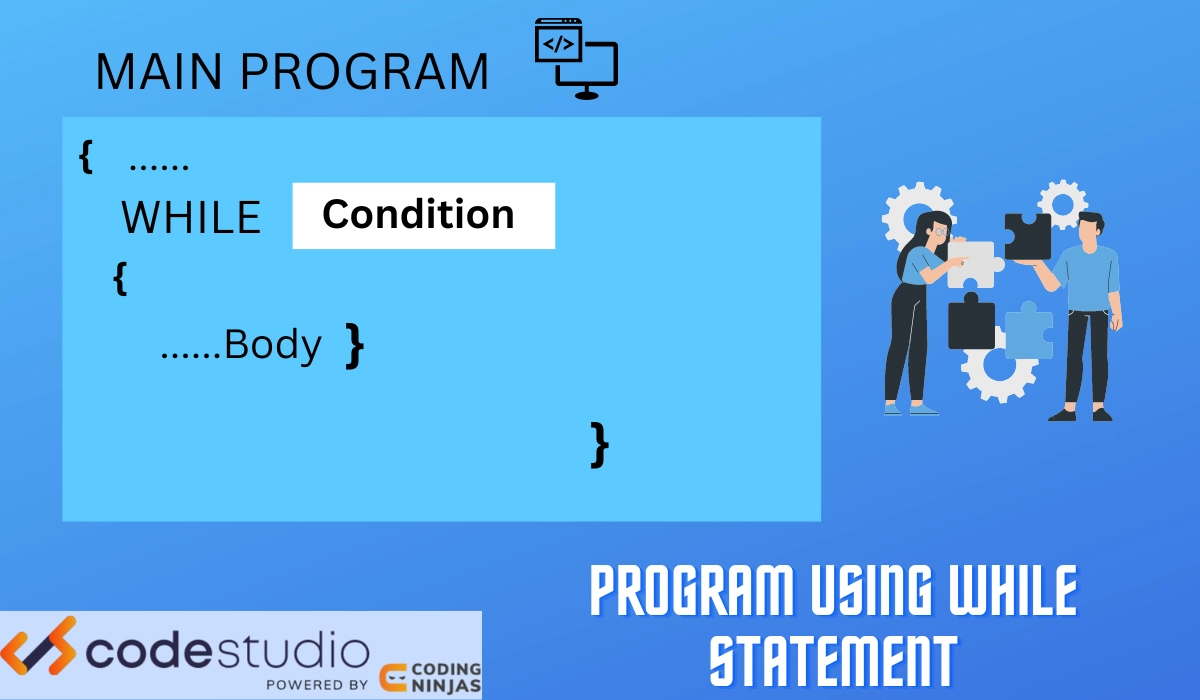### Algorithm

The algorithm for the program is:

• Start.

• Declare variables.

• Print value on screen.

• Set and check the condition under a while.

• Evaluate the sum by adding the initial sum and digit.

• Divide the number by 10.

• Execute the code block inside the statement till the condition passes.

• Print the sum of digits in C on the screen.

• End.

Let’s look at the code below to find the sum of digits in C using the while statement.

### Implementation

``````#include <stdio.h>

// Main body of the program
int main()
{
// Declare the variables
int number, sum = 0;

printf("Enter the number ");
scanf("%d", &number);

printf("The number is = %d\n", number);

// Loop using a while statement
// The loop will run till the number is not equal to 0
while (number != 0)
{
// Sum plus the number divided by 10 to skip a place
sum += number % 10;

// Number is divided by 10
number = number / 10;
}

// Print the sum of the number
printf("Sum: %d\n", sum);

return 0;
}``````

#### Input

``6789``

#### Output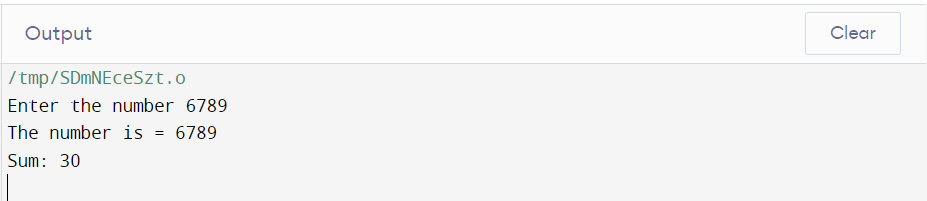### Program Using Char Input

Program using character as an input and While Statement Without Modulus Operator to find the sum of digits of a number in C. It is similar to the example above, but we don’t use the modulus operator (%) here. We can use looping to find the sum of digits of a number in C.

For this example, we will use while statements. While the statement has a condition that determines when the loop is to be terminated. It contains a body that runs till the condition is satisfied. We take the input as a character and convert the data type of the input number into an integer while programming execution.

The picture below shows how the program is executed.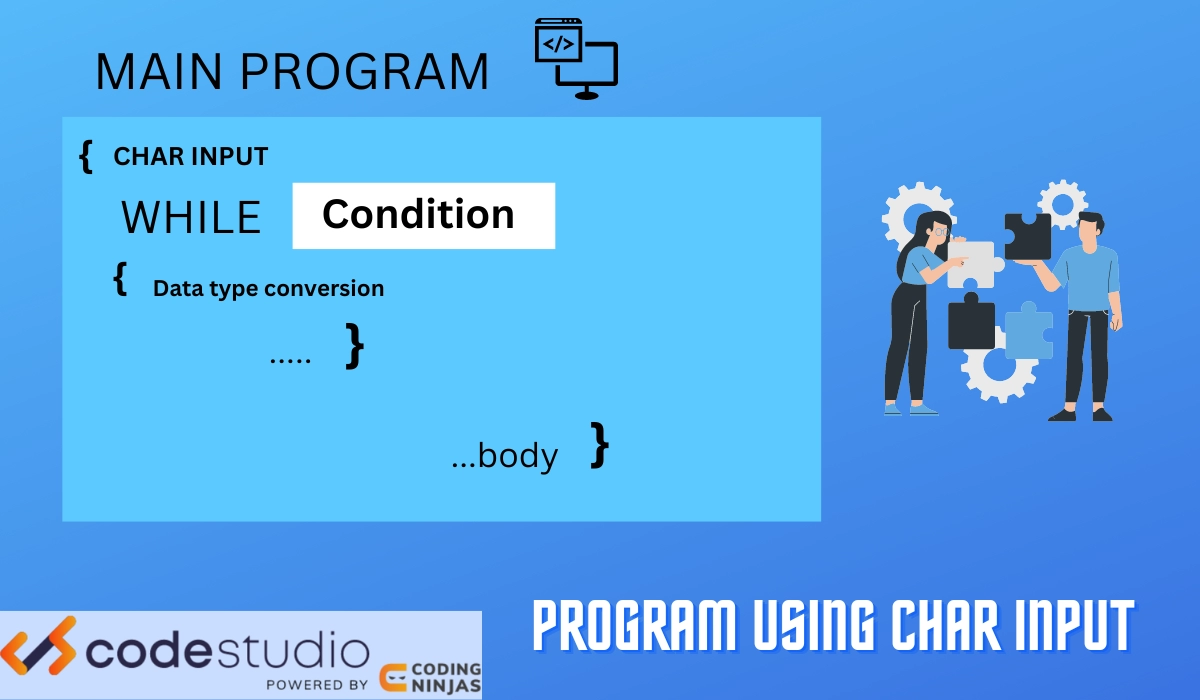### Algorithm

The algorithm for the program is:

• Start.

• Declare number as char input.

• Accept number input from the user.

• Set and check the condition for a while.

• Perform data type conversion from char to int.

• Evaluate the sum by adding the initial sum and digit.

• Increment char number input.

• Execute the code block inside the statement till the condition passes.

• The print sum of digits in C on the screen.

• End.

Let’s look at the code below to find the sum of digits in C using the while statement with character input.

### Implementation

``````#include <stdio.h>

int main()
{
// Declaring variable names
int ch, sum, x;
char num;

// Take number input from the user
printf("Enter a number\n");
scanf("%s", num);

sum = ch = 0;

// Loop using a while statement
while (num[ch] != '\0') {

// Convert character input into an integer
x  = num[ch] - '0';
sum = sum + x;

// Increment of character input
ch++;
}

printf("Sum of digits of %s = %d\n", num, sum);
return 0;
}``````

#### Input

``6789``

### Program Using Recursion

Using recursion functions, we can also perform the sum of a number's digits in C. To understand recursion functions, you need to understand how functions work. We develop a few lines of code to perform a particular action or task. We then group this code block together and give it a name. We can call this name throughout the main body to perform the action listed in the code block.

When we call out the function inside the function itself, it is called a recursive function
The picture below shows how the program finds the sum of a number's digits in C.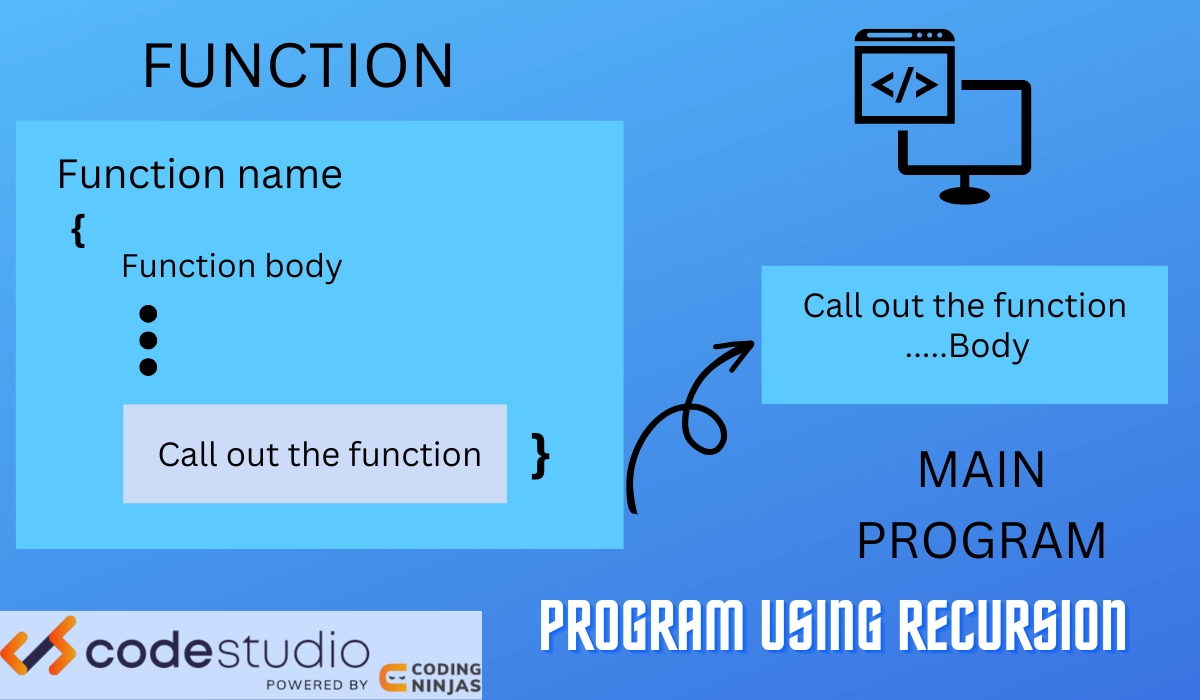### Algorithm

The algorithm for the program is:

• Start.

• Declare function and variables.

• Accept number input from the user.

• Call out the function.

• The print sum of digits in C on the screen.

• Define function.

• Create a base class under the if statement.

• Create a recursion function under the else statement.

• End.

Let’s look at the code below to find the sum of digits in C using the recursion function.

### Implementation

``````#include <stdio.h>

// Declaring a function
int digits_sum(int);

int main()
{
// Declaring variables
int number, result;

// Input from the user
printf("Enter a number\n");
scanf("%d", &number);

// Calling out the function
result = digits_sum(number);

printf("The sum is %d\n", result);

return 0;
}

// Defining the function
int digits_sum(int number) {

// Base case
if (number == 0)
return 0;

// Recursive function
return (number%10 + digits_sum(number/10));
}``````

#### Input

``6789``

Output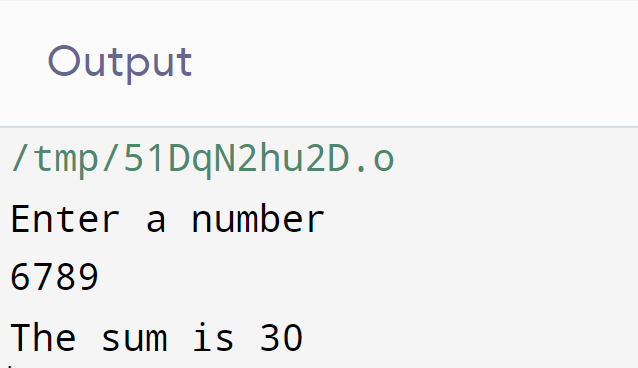### Program Using Loop

We can use looping to find the sum of digits of a number in C. For this example, we will use “for” statements. For statement has three condition that determines the following:

• Starting condition of the loop.

• Termination condition of the loop.

• Increment condition of the loop.

It also contains a body that runs till the condition is satisfied. We use the modulus operator (%), which returns the remainder in this program.
NOTE: when we divide two integers in C language, the result is always an integer.
The picture below shows how the program is executed.

### Algorithm

The algorithm for the program is:

• Start.

• Declare variables.

• Accept number input from the user.

• Use the “for” loop.

• Skip places in the number using the modulus operator.

• Evaluate the sum by adding the initial sum and digit.

• Print the sum of digits in C on the screen.

• End.

Let’s look at the code below to find the sum of digits in C using the For loop statement.

### Implementation

``````#include <stdio.h>
int main()
{
// Declare the variables
int number, sum = 0, x;
printf("Enter a number\n");

// Loop using for
for (scanf("%d", &n); n != 0; n = n/10) {

// Skip the place by tens using modulus
x = n % 10;

// Sum of the digits
sum = sum + x;
}

// Print the output on the screen
printf("Sum of digits of a number = %d\n", sum);
return 0;
}``````

#### Input

``6789``

#### Output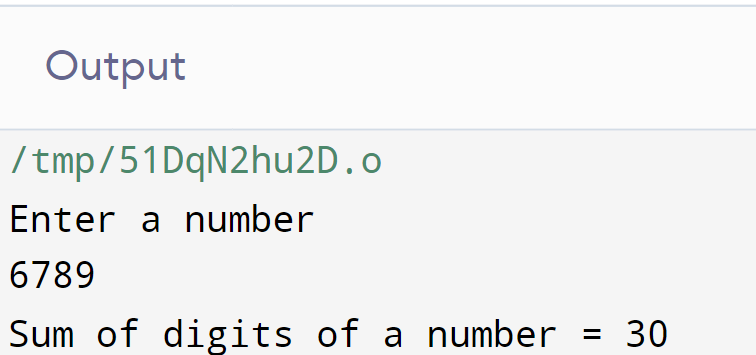## Sum of Numbers in C

As discussed earlier in this article, there is a difference between the sum of digits of a number in C and the sum of numbers in C.
We will now discuss how to get the sum of numbers in C by two simple methods.

### Program with Declared Values

We will derive the sum of two numbers given as a value by the programmer.

### Implementation

``````#include <stdio.h>

// Main function for the body of the program
int main()
{
// Declaring variables as integer
// Give values during the declaration
int n1=5, n2=7, sum;

// Expression for calculating the sum
sum = n1 + n2;

// Expression for printing output on the screen
printf("The sum of the two numbers is %d”, sum);
return 0;
}``````

### Explanation

Let’s understand the steps of the above code step by step-

✏️ In this program, the programmer enters two integers. These two integers are stored in variables n1 and n2, respectively.

✏️ We created a variable called sum, which will be used to store the data after adding two numbers.

✏️ Then, these two numbers are added using the + operator, and the result is stored in the sum variable.

✏️ Finally, the printf() function displays the sum of numbers.

### Program with Input at Run time

We will derive the sum of two numbers taken as input from the user. We will use scanf() for this purpose.

### Implementation

``````#include <stdio.h>

int main()
{
// Declare the variables
int n,m, sum;

// Print a statement for a user to enter the two numbers
printf("Enter two numbers A and B : \n");

// Read and store input numbers from the user as n and m
scanf("%d %d", &n,&m);

// Calculate the addition of n and m
// Using '+' operator
sum = n+m;

// Print the sum
printf("Sum of n and m is: %d", sum);

return 0;
}``````

#### Input

``````Enter two numbers A and B :
2 2``````

### Explanation

Let’s understand the steps of the above code-

✏️ In this program, the user is asked to enter two integers.

✏️ We created a variable called sum, which will be used to store the data after adding two numbers. We also created a variable called n and m as integers for storing number input from the user.

✏️ Then, the expression is set to add two numbers using the + operator, and the result is stored in the sum variable.

✏️ The inputs are scanned with the scanf() function and stored in variables n and m.

✏️ It then adds the variables A and B using arithmetic operators and stores the result in the variable sum.

✏️ Finally, the printf() function displays the sum of numbers.

### How to add two numbers in C?

Additional problems in C can be done by simple mathematical reasoning, just keeping in mind gathering basic syntaxes.

### What does %d mean in C?

It means we are printing integer values.

### Can we solve the same problem in subtraction and other mathematical operators?

Yes, we can solve the problem by changing the operator sign and other statements to change.

### Which coding language is mainly required in companies?

Most companies ask for JAVA, CPP, and Python (for machine learning, deep learning, etc.)

### Is C++ harder or C?

C++ has new features compared to C. C can be considered the primary language to learn, whereas C++ includes some new classes and features different from C.

## Conclusion

We have studied how to perform the sum of digits in C using various methods. We have also learned how to derive the sum of two numbers. We have observed basic syntaxes here, along with codes. This article deals with elementary programs, so they help us build our logical skills. So ninjas practice more and more such programs here, C language.
Refer to the Below topics for more understanding.

Please refer to our guided paths on Codestudio to learn more about DSA, Competitive Programming, JavaScript, System Design, etc. And also, refer to the mock test and problems available. Have a look at the interview experiences and interview bundle for placement preparations.

Happy Learning!